6.10 Geometric Solid Figures:

6.10.1 Properties of solid figures

In science you have learnt differences between solids, liquids and gasses. You also know to draw some geometrical figures (polygons) on

a plane (called 2 dimensional). Geometrical figures which are solids in shape are cuboids, cube, cylinder, cone, sphere, etc.

Differences between planes and solid figures are:

 No Plane figures Solid figures 1 They only have areas They occupy space and hence have volume 2 Measured by length and breadth Measured by length and breadth and height 3 They are formed by a minimum of 3 Coplanar (on same plane) points They are formed by a minimum of 4 points on different planes

Properties of Cuboids:

 1. They have eight vertices (A,B,C,D,E,F,G,H) 2. They have six faces [bottom (EFGH), top(ABCD) and 4 sides(ABFE,BCGF,DCGH,ADHE) 3. All sides are rectangular in shape 4. They are formed by 12 line segments (edges)   Four vertical sides of cuboids are called ‘lateral surfaces’(ABFE,BCGF,DCGH,ADHE) and the other two are called ‘base’(EFGH) and ‘top’(ABCD). The lateral surface area of the cuboid = Area of ABFE + Area BCGF, Area of DCGH + area of ADHE  = lh+bh+lh+bh = 2h(l+b)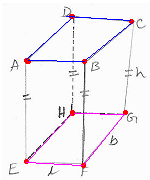Have you seen houses, office buildings, memorials and other constructions in shapes other than Quadrilaterals? You must have thought how

they calculate the areas of these constructions, for buying paints and other materials. Similarly people construct water tanks in shapes other

than cuboids for various reasons. Is it not necessary for us to calculate the volume of these solids to know the capacity of these tanks?

Here we will be studying few such solid figures.

Since cuboids have rectangle as bases, let us look at other types of solids whose bases are polygons (triangle, pentagon and hexagon).

Such figures are called ‘Prisms’.

 ‘Prism’ can be defined as a closed solid figure with rectangular lateral surfaces  And with parallel and congruent (matching in all aspects) polygons as base and top.   Properties of prisms are: 1. All the lateral surfaces are rectangular 2. Base and top are of same shape and size    The line where two lateral surfaces intersect is called  ‘edge’   Depending on the type of  the base of the prism we have ‘Triangular prism’, ‘Rectangular prism’, ‘Pentagonal prism’, ‘Hexagonal prism’, etc…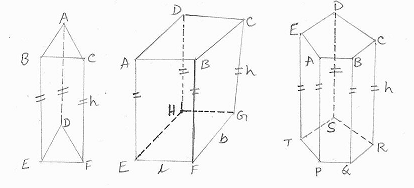What if the prisms do not stand straight on a plane? Let us look at the figures drawn below.

In the first figure, base plane and top plane are perpendicular to each other and the height of this prism is the edge. In the second figure,

base plane and top plane are parallel but the edges are not perpendicular to the base or top. That is why we cannot directly measure the

height but we can measure the slanted height.

 No Right Prism Right Prism No Oblique Prism Oblique Prism 1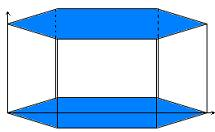The edges of the base are perpendicular to the plane of the base The edges of the base are not perpendicular to the plane of the base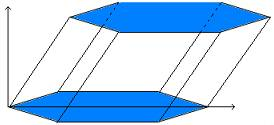2 The lateral surfaces are rectangles The lateral surfaces are parallelograms 3 The edge is height of the prism The edge cannot be taken as the height of the prism as it is not is not vertical

6.10.2 Surface Area of Prisms

Let the Prism ABCDEF be a triangular prism with equilateral triangle as the base.

Observe the following:

 1. The base ABC and top DEF are equilateral triangles with sides ‘a’ 2.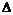ABC andDEF are parallel and congruent 3. The lateral edges AE, BF and CD are parallel and are of equal height ‘h’ 4. The 3 lateral surfaces are ABFE, BCDF and ACDE. Area of each surface = a*h=ah Since this prism has 3 lateral surfaces, lateral surface area = 3ah. Total Surface Area (TSA) of the prism = area of base + area of top + area of lateral surfaces = 2*(area of base) + 3ah (because area of base = area of top) Perimeter of the base (P) is 3a (sum of 3 sides of the equilateral triangle). Thus TSA = 2*area of the base of prism + (perimeter of the base)*height = 2B+Ph Where ,B = Area of the base of the prism ,P = Perimeter of the base of the prism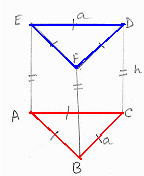The above formula for Total Surface Area is applicable to any right prism.

6.10.2 Problem 1: Find the altitude of right prism whose lateral surface is 143sqcm and perimeter of the base (P) is 13cm.

Solution:

We know that the formula for lateral surface area = Ph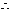143 = 13h and hence h = 11cm.

6.10.2 Problem 2: The base of a square based prism has edge equal to 2cm and height 12cm.

A hole extending from top to base is in the shape of an equilateral triangular prism with an edge

of length 1cm.  Find out the total surface area of the solid (exterior painting area of the prism).

Solution:

 Method: First we need to calculate the TSA of the solid without the hole. From this TSA we need to subtract the area (both base and top) of the hole, to get the area of painting. Since the prism is a square prism of side 2cm; the area of the base = 4sqcm Perimeter of the base (P) is 8cm Total Surface Area (without considering the hole) = 2B + Ph =8+96=104sqcm The hole is of the shape of an equilateral triangle of side 1cm and hence the area of the base/top = (12 *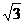)/4 =/4sqcm   Therefore TSA after considering the hole = TSA of the solid without the hole – area of the base of the hole – area of the top of the hole = 104 -/4-/4 = (104 -/2)sqcm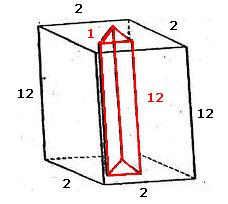Note: We have used the following formula to calculate the area of an equilateral triangle. Area of triangle = bh/2 (where b is the base of the triangle and h is the height of the triangle) In the case of an equilateral triangle, h = b*()/2(Use Pythagoras theorem to calculate the altitude of the right angled triangle with base = half the height of hypotenuse: b2=height2+(b/2)2): height2= 3b2/4: height = b*/2 Therefore Area of an equilateral triangle = (b2*)/4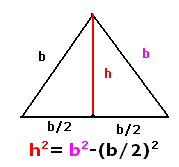6.10.3 Pyramids

We called a solid, with polygons as base and top and with rectangle as lateral surfaces, a Prism. What if we have a solid figure without a top

but with its sides converging at a point?

 Definition: A pyramid is a solid figure with polygonal base and triangular sides (lateral surfaces) converging at a point.   The converging point is called ‘vertex’. Since sides of a pyramid converge at a point, pyramids do not have top surface.     Depending on the type of polygonal base of the pyramid, we classify pyramids as ‘Triangular pyramid’, ‘Rectangular pyramid’ and ‘Hexagonal pyramid’ as shown in the figures on  right hand side.   In the adjacent figures, P is the vertex and ABC, ABCD, ABCDEF are base of the pyramids.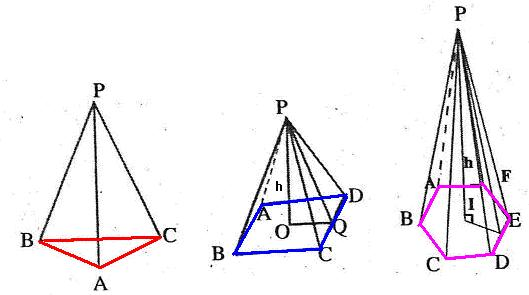The relationship between base, height and slant height in a square pyramid:

 Let the side of base of pyramid      =b Let the height of the pyramid        =h Let the slant height of the pyramid =l   Then by Pythagoras theorem   l2= (b/2)2+ h2l =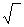{(b/2)2+ h2}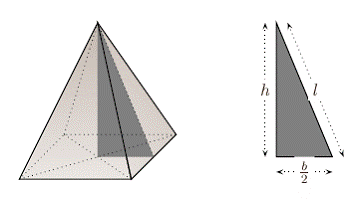Surface area of a pyramid

 PABCD is a square pyramid. ABCD is the base and it is a square. Q is the midpoint of ABCD. PQ is the height (h) and N is the mid point of the edge BC. PN is the slant height (l). AB=BC=CD=DA=a PAB, PBC, PCD and PAD are the 4 lateral surfaces which are congruent isosceles triangles. Perimeter of the base (p) = 4*length of base 1. Lateral surface area of the square pyramid PABCDP: = sum of the areas of 4 congruent lateral surfaces (PAB, PBC, PCD, PAD) = 4*(area of one triangle) = 4(1/2) base*slant height)) = 4al/2 = 2al = (1/2)(perimeter of base)*(slant height)   Total Surface Area (TSA) of square based pyramid = Area of the base + Lateral surface area = a2+ 1/2 (pl)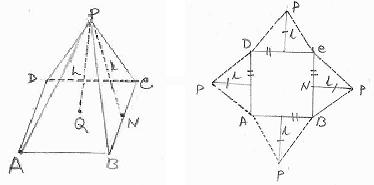2. Surface area of a regular triangular pyramid PABC:

 ABC is an equilateral triangle (AB=BC=CA=a). Let N be the mid point of the side BC and hence PN is the slant height (l). PAB, PBC and PCA are 3 lateral surfaces which are congruent isosceles triangles. Perimeter of the base (p) = 3*length of base   The lateral surface area of a regular triangular pyramid  = sum of the areas of 3 congruent lateral surfaces (PAB, PBC,PCA) = 3*(area of one triangle) = 3(½ base*slant height) = 1/2 (3al) = 1/2 (Perimeter of base)*(slant height)= 1/2 (Pl)   Total Surface Area (TSA) of a regular triangular pyramid = Area of the base + Lateral surface area = B+ 1/2 (Pl)     Since base is an equilateral triangle, B = (a2)/4(Refer 6.10.2 problem2)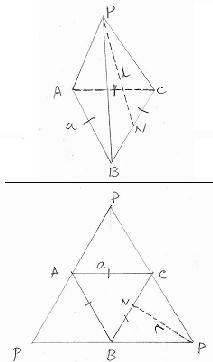6.10.3 Problem 1: A pyramidal tent with a square base of side 5m and slant height of 4m is put up for a function and is covered by a canvas.

If the rent for usage of tent is paid based on the area of canvas, find out the area of canvas.

Solution:

 Here P = 4*5 m=20m     l = 4 m Lateral surface area = ½(Pl) = ½(20*4) = 40sqmts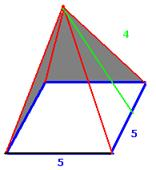6.10.3 Problem 2: Total surface area of a square based pyramid is 125sqcms and the perimeter of the base is 20cm. find the area of the

base and slant height

Solution:

 Given: Perimeter {p=4a} of the square is 20cm. Hence the side (a) is 5cm. Area of the base (B=a2) = 25sqcm   Since TSA = Area of the base +½(Pl).  On substitution we get 125 = 25+½(Pl): 125=25+½(20*l): 125 = 25+10*l 10*l = 125-25 =100 (transposition)   Thus the slant height (l) = 10cm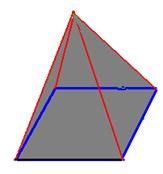6.10.4 Volume of Prisms and pyramids

 We know that the volume of a cuboid = length*breadth*height.   We also know that (length*breadth) gives the area of the base of the cuboid.   So, Volume of cuboid = (Area of base) * height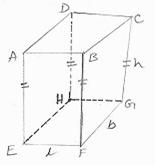Volume of a ‘triangular right prism’

 We can think 2nd figure as 2 equal parts of the first figure cut in the middle. Hence the volume of square based prism is twice the volume of triangular based right prism. We also notice that the square base is made up of two identical isosceles triangles. Volume of a triangular right prism = 1/2 (Volume of square based prism) = 1/2 (‘base area of square based prism’*height) = 1/2 (2*‘area of triangular base’*height) = the area of triangular base’*height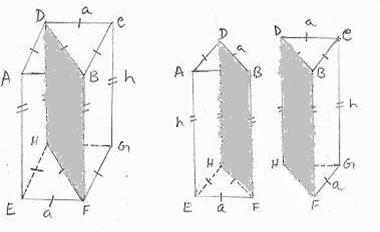In general, volume of a prism is equal to the product of the area of its base and its height.

6.10.4 Problem 1: The volume of a regular triangular based prism is 27cc and its height is 12cm. Find the length of side of the base.

Solution:

 Let ‘b’ be the length of side of the base. We know that the area of equilateral triangle = (b2)/4 As per the formula:Volume of the prism = ‘base area’ * height = (b2)/4* height. On substituting values we have 27= (b2)*12/4. On cancelingon both sides, we have 27 = 12 *b2/4 3 b2=27 :b2=9 :b=3cm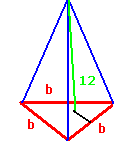Volume of a pyramid

 Let ABCD be the square base (of side ‘a’ units) of a pyramid PABCD with P as vertex. From P, drop a perpendicular to the base to meet the base at M. From the figure it can be seen that PM = h = a/2.  From the cube(refer to adjacent figure) PABCD, we can totally make 6 congruent( identical) pyramids PABCD, PBCC1B1, PCC1D1D, PC1D1A1B1, PD1A1AD and PABB1A1. (Each of these pyramid’s base corresponds to each face of the cube. We can say that when these 6 pyramids are joined, we will have a square based pyramid.)   As can also be visualized from the figure on the right hand side Volume of square based pyramid  = 1/6(volume of cube)  = 1/6{(Area of base) * a}  = 1/6{(Area of base) * 2h} (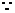h=a/2)  = 1/3{(Area of base) * h}  = 1/3 (Bh); where B is area of the base                     = 1/3 volume of square prism of same base and height.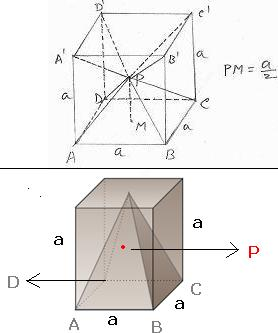Similarly we can prove that volume of a triangular pyramid is one third the volume of prism.

In general, volume of a pyramid is equal to one third the product of its base area and its height.

6.10.4 Problem 2:  A tent of pyramidal shape is made out of canvas. It occupies a square area of 100sqmts on the ground and the

slant height of the pyramid is 13mts. Calculate the air space inside the tent. (To get the actual height (h) of the tent,

use the formula  h2= l2-(a/2)2  where l is the slant height and a is the length of base )

Solution:

 Since base area is 100sqmts, a=10m; l is given to be 13 m. We need the vertical height of the tent to calculate air space. We are given h2 = l2-(a/2)2; on substitution we have h2 = 169-25 = 144h=12m.   Air space inside the tent = Volume of the tent = 1/3Bh = 1/3(100*12) = 400cu.mts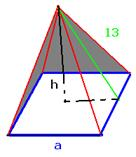6.10.4 Problem 3: A farm has a water tank of dimensions (l=4m, b=1.2m and h=1m) for cows to drink water and  is filled with water once

in the morning. If a cow consumes 40lts of water in a day, find out how many cows would be able to drink water on a given day

(note 1cu.mts = 1000Litres (lts))

Solution:

Volume of tank = 4*1.2*1cu.mts = 4.8cu.mts = 4800Litres (1cu.mts = 1000Litres)

Number of cows that can drink water = 4800/40 = 120 cows

6.10.4 Problem 4: Find the height of a pyramid whose volume is 900cu.mts and base is a rectangle of sides 20m and 15m.

Solution:

 We know that volume of a pyramid = 1/3 (Bh) (where B is the area of base and h is the height) On substituting values for volume and B, we have 900 = 1/3(20*15)h; 900 = 100h; h =9m Thus the height of the pyramid is 9m.6.10.4 Problem 5: A brick’s length, breadth and height is 18,12 and 3 inches respectively. A wall’s length,width and height is 8,5 and 3Hastas’ (A measuring unit). Find the volume of the wall, the number of bricks in the wall and the number of rows of bricks in the wall (Lilavati Shloka 227)

 Volume of wall = 8*5*3=120 cubic Hastas Voume of brick = (18*12*3)/(24*24*24) = (3/64) cubic Hasta (  1 Hasta = 24 inches) Number of bricks = 120*(64/3) = 2560 Number of rows of bricks = 3*24/3 = 24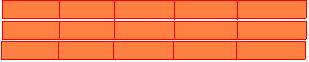6.10 Summary of learning

 No Points to remember 1 Total surface area of a cuboid is 2(lb+bh+lh)  and its lateral surface area = 2(bh+lh) 2 Prism is a closed solid figure with rectangular lateral surfaces and with parallel and congruent polygons as base and top. 3 Total Surface Area of a Right Prism = 2*area of the base of prism + (perimeter of the base) * height 4 A pyramid is a solid figure with polygonal base and triangular sides (lateral surfaces) converging at a point. The converging point is called ‘vertex’. 5 Total Surface Area of square based pyramid = Area of the base + Lateral surface area= a2+ 1/2(pl) where p is perimeter of the base and l is the slant height 6 Total Surface Area  of regular triangular pyramid = Area of the base + Lateral surface area= B+ 1/2(Pl) where P is perimeter of the base and l is the slant height 7 Volume of a cuboid is equal to the product of its length, breadth, and height 8 Volume of a prism is equal to the product of its base area and its height. 9 Volume of a pyramid is equal to one third the product of its base area and its height.